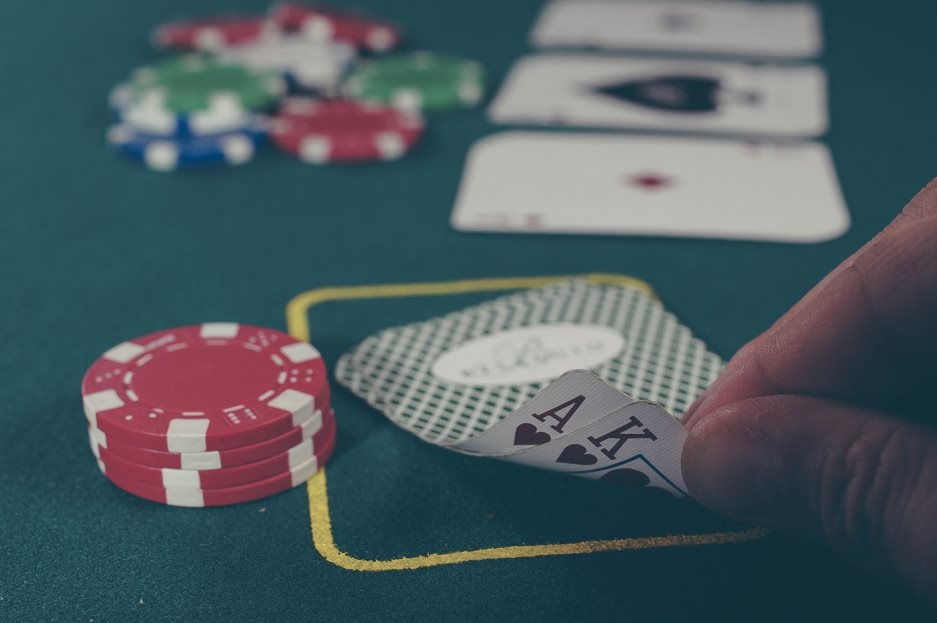# Independent and Dependent Events – Understanding Independent and Dependent Events in Probability

Probability, a fascinating branch of mathematics, helps us understand the likelihood of an event occurring. Within probability the concepts of independent and dependent events are pivotal. These two notions are key in defining and comprehending the interrelationships between various events.In this Blog Post we will learn:
1. Independent Events
2. Dependent Events
3. Key Distinctions
4. Conclusion

Let’s dive deeper into these concepts and understand them with examples.

## 1. Independent Events

Definition: Two events, A and B, are independent if the occurrence of one event does not influence the occurrence of the other.

Probability Rule for Independent Events: If $A$ and $B$ are independent events, the probability of both $A$ and $B$ occurring is the product of their individual probabilities.

$$P(A \text{ and } B) = P(A) \times P(B)$$

Example: Tossing a coin and rolling a dice.

Let’s consider the event A to be getting a head on a coin toss and event B to be rolling a 5 on a die.

• $P(A) = \frac{1}{2}$ (since there are 2 possible outcomes: head or tail)
• $P(B) = \frac{1}{6}$ (since there are 6 possible outcomes on a die)

If we toss a coin and roll a die simultaneously, the occurrence of a head doesn’t affect the outcome of the die roll. Thus, the probability of getting both a head and rolling a 5 is:

$$P(A \text{ and } B) = P(A) \times P(B) = \frac{1}{2} \times \frac{1}{6} = \frac{1}{12}$$

## 2. Dependent Events

Definition: Two events, $A$ and $B$, are dependent if the occurrence of one event influences the occurrence of the other.

Probability Rule for Dependent Events: If $A$ and $B$ are dependent events, the probability of both $A$ and $B$ occurring is:

$$P(A \text{ and } B) = P(A) \times P(B|A)$$

Where $P(B|A)$ is the probability of $B$ occurring given that $A$ has already occurred.

Example: Drawing cards from a deck without replacement.

Let’s say event $A$ is drawing an Ace from a standard deck of 52 cards, and event $B$ is drawing another Ace right after the first one without returning the card back into the deck.

• $P(A) = \frac{4}{52}$ or $\frac{1}{13}$ (since there are 4 Aces in a deck)
• If the first card drawn is indeed an Ace, there are now only 3 Aces left and the total number of cards has reduced to 51. Thus, $P(B|A) = \frac{3}{51}$ or $\frac{1}{17}$.

Given these probabilities, the likelihood of drawing two Aces in succession without replacement is:

$$P(A \text{ and } B) = P(A) \times P(B|A) = \frac{1}{13} \times \frac{1}{17} = \frac{1}{221}$$

## 3. Key Distinctions

1. Interdependence: While the outcomes of independent events are not influenced by one another, the outcome of one dependent event does impact the probability of subsequent events.
2. Calculation: With independent events, you simply multiply the individual probabilities. However, for dependent events, one must account for the changed conditions after the first event occurs.

## 4. Conclusion

Understanding the difference between independent and dependent events is crucial in many real-world situations, from gaming strategies to complex statistical analysis in research. Recognizing and correctly applying these concepts will provide a strong foundation for further exploration into the rich and vast world of probability.

## Similar Articles

### Logistic Regression – A Complete Tutorial With Examples in RCourse Preview

## Machine Learning A-Z™: Hands-On Python & R In Data Science

### Free Sample Videos:#### Machine Learning A-Z™: Hands-On Python & R In Data Science#### Machine Learning A-Z™: Hands-On Python & R In Data Science#### Machine Learning A-Z™: Hands-On Python & R In Data Science#### Machine Learning A-Z™: Hands-On Python & R In Data Science